Equable Trirectangular Tetrahedron

EQUABLE TRIRECTANGULAR TETRAHEDRON

Balmoral Software

 Solutions: 6 (integer dimensions) Solutions: 3 (non-integer dimensions, conjectured)

A trirectangular tetrahedron is a four-sided polytope created from a corner of a cuboid: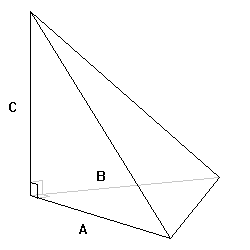There are exactly 6 equable trirectangular tetrahedra with integer dimensions. It is conjectured that no more than 3 additional equable trirectangular tetrahedra have non-integer dimensions.

Let the lengths of the three orthogonal edges meeting at the corner be A, B and C; these are referred to as the dimensions of the tetrahedron. We begin by showing that the dimensions are rational. The volume of the tetrahedron and the areas of the three non-base faces are integers by convention:

ABC/6, AB/2, AC/2, BC/2 ∈ ℤ
It follows that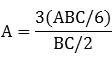is rational, and analogously for B and C. Therefore, we can express A, B and C in terms of their lowest common denominator d as a/d, b/d and c/d, where a, b, c and d are positive integers. Without loss of generality, we may take
a ≥ b ≥ c ≥ 1
Equating volume V and surface area S, we have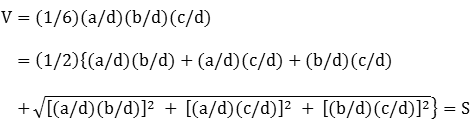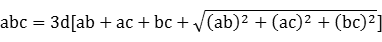There can be no equable solution when the shortest edge length c/d ≥ 15: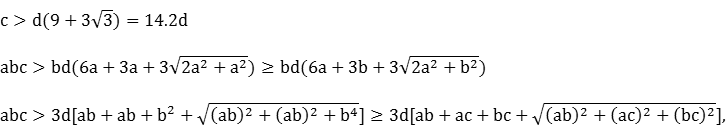which shows that  is not satisfied since the volume exceeds the surface area. Next, assume that c ≤ 6d. Then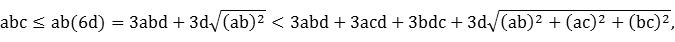so again  is not satisfied. Therefore, all equable solutions occur when
6d + 1 ≤ c ≤ 15d - 1
Assume the above inequality holds, and let b > 12d(15d - 1). Then
[a - 6d(15d - 1)][b - 6d(15d - 1)] ≥ [b - 6d(15d - 1)]2 > [6d(15d - 1)]2
and it follows that
abc ≥ ab(6d + 1) > 6abd + 6ad(15d - 1) + 6bd(15d - 1) ≥ 6abd + 6acd + 6bcd

= 3d(ab + ac + bc) + 3abd + 3acd + 3bcd

Since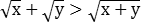for positive x and y, we have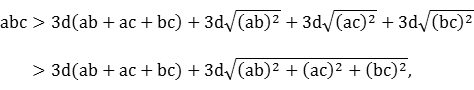which shows that  is not satisfied. Therefore, all equable solutions occur when
 6d + 1 ≤ c ≤ 15d - 1 and c ≤ b ≤ 12d(15d - 1)

Dividing both sides of  by 3abcd, we have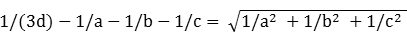The left side must be positive for an equable solution. Squaring both sides reduces to
 [(b - 6d)(c - 6d) - 18d2]a = 6d(b - 3d)(c - 3d) - 54d3 
Since b ≥ c ≥ 6d + 1, the right side is positive. The condition
 (b - 6d)(c - 6d) > 18d2 
must hold so that division in  produces a positive value for the edge length numerator a: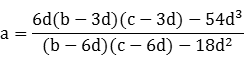By convention, the lateral face areas ab/(2d2), ac/(2d2), bc/(2d2) and the volume abc/(6d3) must all be integers. By subtraction, the base area is also an integer since the total surface area is an integer.

For a fixed value of d, it is a simple matter to evaluate the

(9d - 1)(360d2 - 45d + 2)/2
feasible values of (b,c) in  to find those for which:
•  is satisfied
• an integer value for a is produced by 
• a ≥ b (to omit duplicates)
• ab/(2d2), ac/(2d2), bc/(2d2) and abc/(6d3) are integers
• gcd(a,b,c,d) = 1 (to omit duplicates due common factors in fractions)
When d = 1, a search is performed over 1268 values of (b,c), which results in six solutions with all integer edge lengths. When the lowest common denominator is 2, there are 11,492 (b,c) values to search, but none produce additional answers satisfying the above criteria. When d = 3, a search of 40,391 value pairs produces three more solutions, each containing at least one non-integer edge length. It is conjectured that no further solutions exist for d ≥ 4. The 9 known equable trirectangular tetrahedra are as follows, ordered by volume:
dABCFace AB/2Face AC/2Face BC/2BaseV=S
118141212610884186504
124121214414472216576
3361232/321619264296768
132248384128964161024
1541210324270604261080
166188594264726541584
31441228/38646725610962688
116816813446726415043584
33363620/360481120120615213440